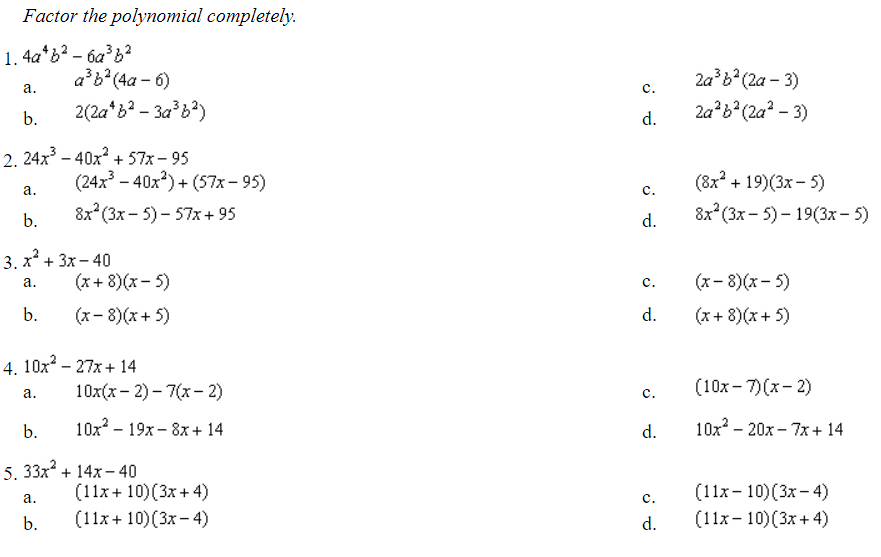# Warmups Topics 5-5Answers (C, C, A, C, D)

Notes:

1. Find the GCF (greatest common factor) of the monomials in the given polynomial, and use it in grouping the polynomial.
2. Group the monomials to find the GCF (greatest common factor), factor the GCF of each binomial, and then use the Distributive Property to obtain the factors.
3. To find the coefficient of the x-terms, find two numbers whose product is 140 and whose sum is 27.
4. To find the coefficient of the x-terms, find two numbers such that their product is 1320 and their difference is 14.

(Source)